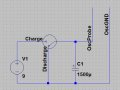# Test capacitor with an oscilloscope?

#### GregJ7

Joined Jun 7, 2014
42
I'm trying to figure out how to use a simple oscilloscope to show me a curve so I can determine if a capacitor is working as it should or not (and then calculate its Farad rating). First I want to make sure I have set the non-oscilloscope portion up correctly. My goal was to use a switch that discharges the cap in one position and applies power to the cap in another position, which in theory will trigger the oscilloscope when the voltage becomes non-zero. Can anyone verify that my schematic will do that? Does the voltage on the hot ("+") end of the cap rise as the cap charges and then stay constant when it is fully charged?#### crutschow

Joined Mar 14, 2008
29,825
You need a resistor in series with the Charge line so that you can see the charge curve on the oscilloscope and calculate the capacitance from the RC time-constant (in one time-constant it charges to 0.636 of the input voltage).
Note that you may need some small resistance in the Discharge line to keep the peak discharge current to a safe level for the switch you are using.

Yes the voltage rises exponentially to the supply voltage and stays there.

•GregJ7